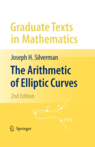# The Arithmetic of Elliptic Curves (2nd Edition)

## Joseph H. Silverman

### Springer-Verlag – Graduate Texts in Mathematics 106 ISBN: 978-0-387-09493-9 – 2nd ed. – © 2009 – 513 + xviii pages Math. Subj. Class: Primary 11G05; Secondary 11D25, 11G07, 14G05, 14H25, 14H52

The Arithmetic of Elliptic Curves is a graduate-level textbook designed to introduce the reader to an important topic in modern mathematics. The theory of elliptic curves is distinguished by its long history and by the diversity of the methods that have been used in its study. This book treats the arithmetic theory of elliptic curves in its modern formulation, through the use of basic algebraic number theory and algebraic geometry. The book begins with a brief discussion of the necessary algebro-geometric results, and proceeds with an exposition of the geometry of elliptic curves, the formal group of an elliptic curve, and elliptic curves over finite fields, the complex numbers, local fields, and global fields. Included are proofs of the Mordell–Weil theorem giving finite generation of the group of rational points and Siegel's theorem on finiteness of integral points.

For this second edition of The Arithmetic of Elliptic Curves, there is a new chapter entitled Algorithmic Aspects of Elliptic Curves, with an emphasis on algorithms over finite fields which have cryptographic applications. These include Lenstra's factorization algorithm, Schoof's point counting algorithm, Miller's algorithm to compute the Tate and Weil pairings, and a description of aspects of elliptic curve cryptography. There is also a new section on Szpiro's conjecture and ABC, as well as expanded and updated accounts of recent developments and numerous new exercises.

The book contains three appendices: Elliptic Curves in Characteristics 2 and 3, Group Cohomology, and a third appendix giving an overview of more advanced topics.

 Contents Algebraic Varieties Algebraic Curves The Geometry of Elliptic Curves The Formal Group of an Elliptic Curve Elliptic Curves over Finite Fields Elliptic Curves over C Elliptic Curves over Local Fields Elliptic Curves over Global Fields Integral Points on Elliptic Curves Computing the Mordell--Weil Group Algorithmic Aspects of Elliptic Curves (new to the 2nd edition) Elliptic Curves in Characteristics 2 and 3 Group Cohomology: H0 and H1 Further Topics: An OverviewClick on the links for the following material. Errata List (last updated October 2022)

## Web Resources

• PARI - free software. PARI contains a large library of elliptic curve functions.
• SAGE - free software. SAGE contains a large library of elliptic curve functions.
• Cremona's Tables - Online table of elliptic curves of small conductor.
• Tables of elliptic curves with high rank:

## Contact Information

No book is ever free from error or incapable of being improved. I would be delighted to receive comments, good or bad, and corrections from readers. You can send mail to me at